CBSE Class 10 Sample Paper for 2018 Boards

Class 10
Solutions of Sample Papers for Class 10 Boards

### The following distribution shows the daily pocket allowance of children of a locality. The mean pocket allowance is 18. Find the missing frequency k.

 Daily Pocket allowance  (in rupees) 11 − 13 13 − 15 15 - 17 17 − 19 19 − 21 21 − 23 23 − 25 Number of Students 3 6 9 13 k 5 4

### The following frequency distribution shows the distance (in metres) thrown by 68 students in a Javelin throw competition.

 Distance 0 − 10 10 − 20 20 − 30 30 − 40 40 − 50 50 − 60 60 − 70 Number of Students 4 5 13 20 14 8 4

### Draw a less than type Ogive for the given data and find the median distance thrown using this curve.

This is a question of CBSE Sample Paper - Class 10 - 2017/18.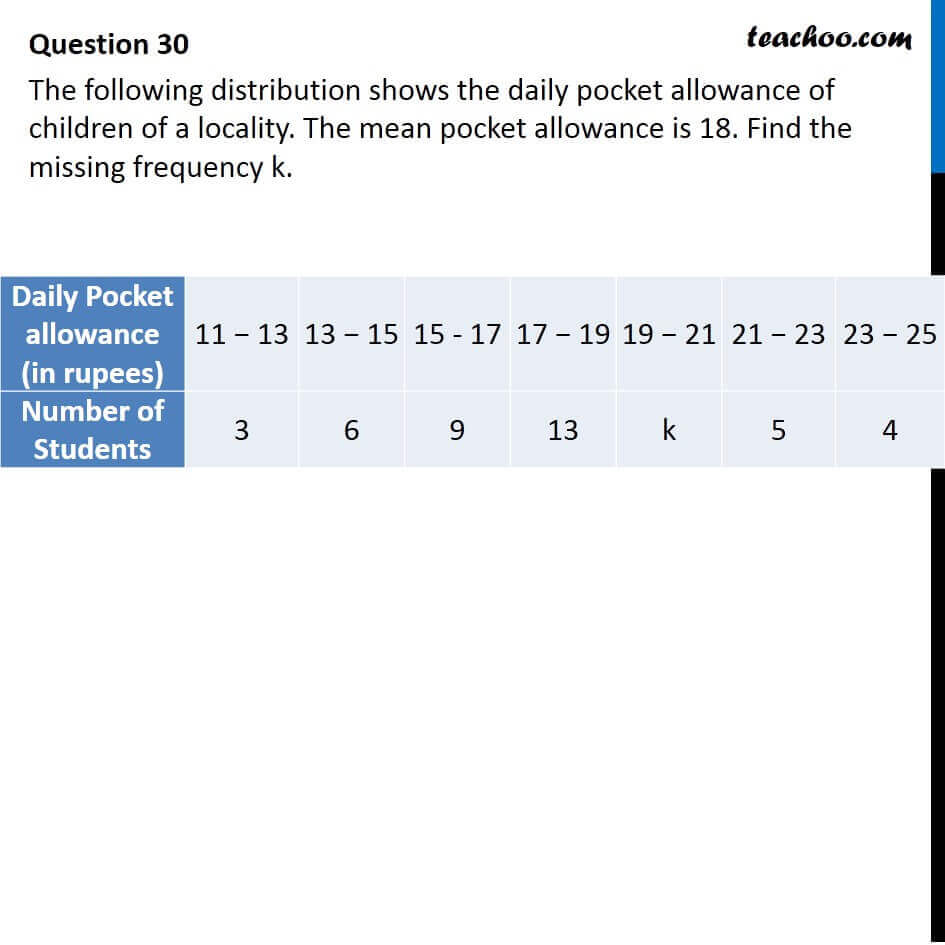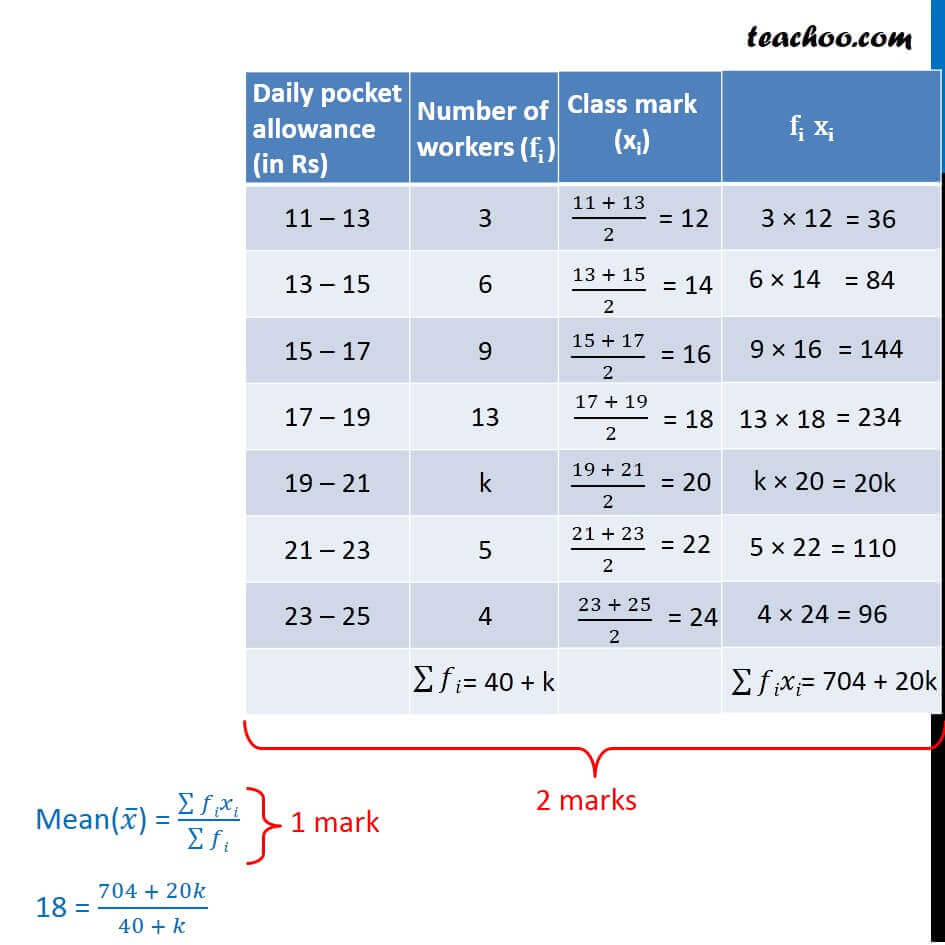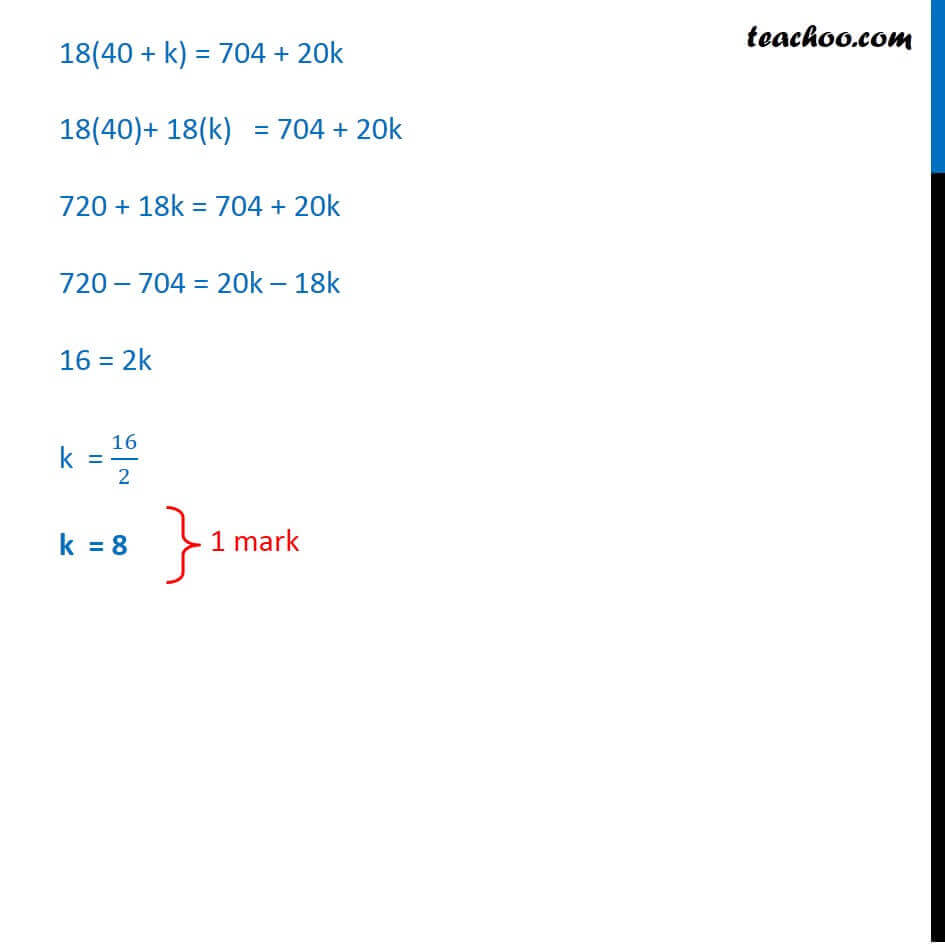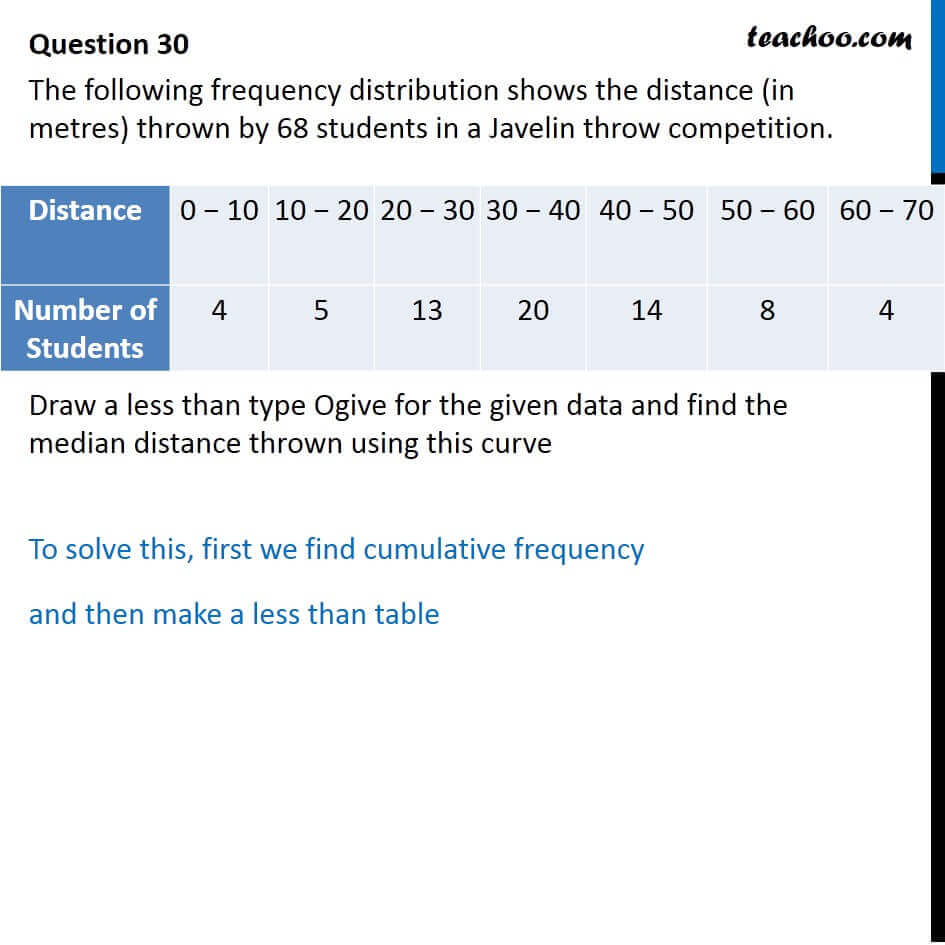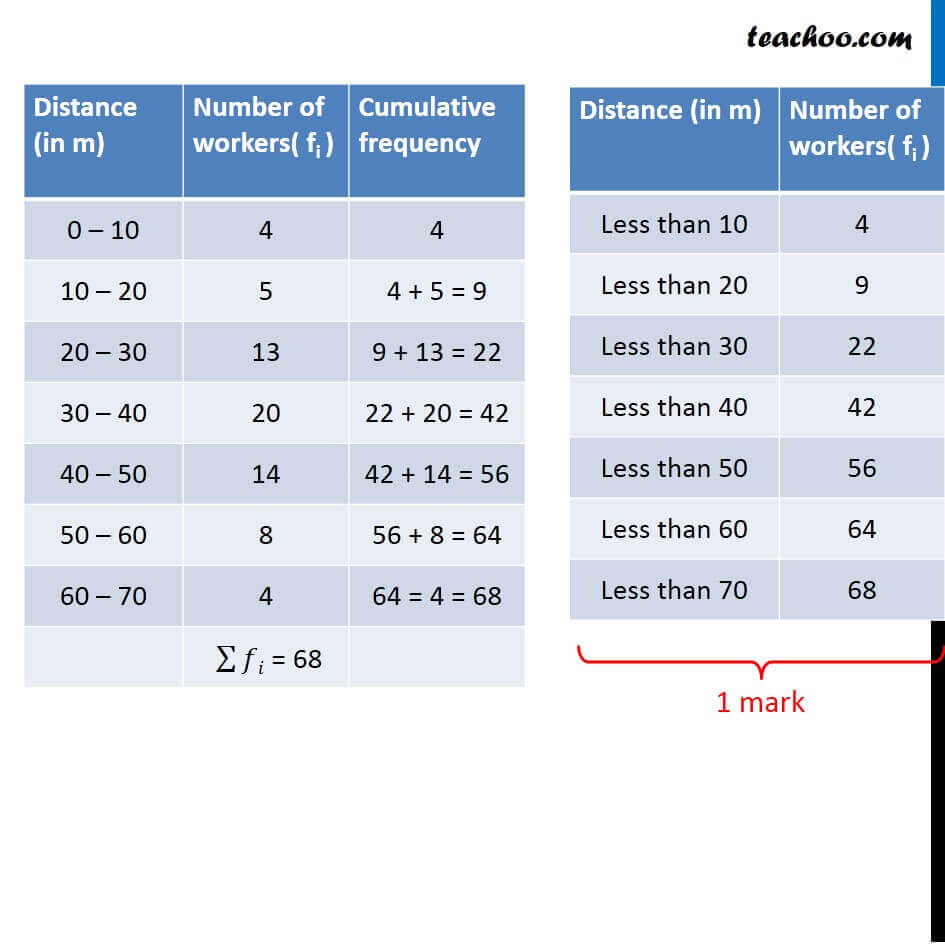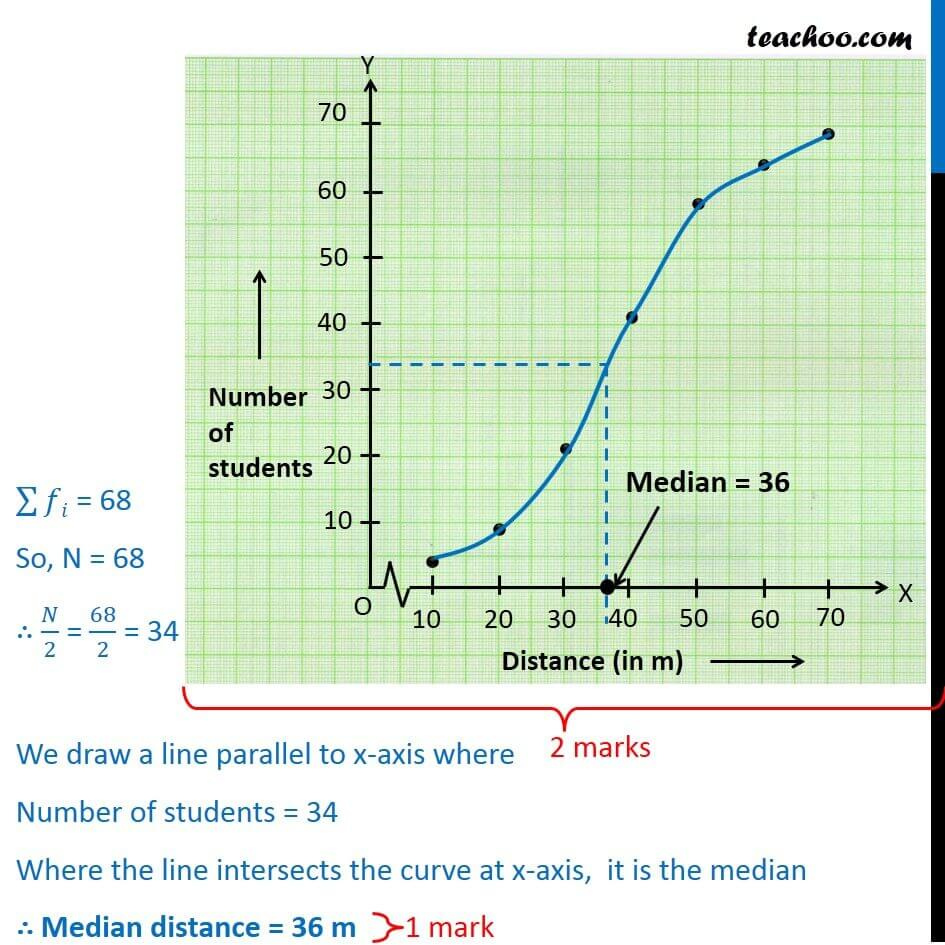Learn in your speed, with individual attention - Teachoo Maths 1-on-1 Class

### Transcript

Question 30 The following distribution shows the daily pocket allowance of children of a locality. The mean pocket allowance is 18. Find the missing frequency k. Daily Pocket allowance (in rupees) 11 − 13 13 − 15 15 - 17 17 − 19 19 − 21 21 − 23 23 − 25 Number of Students 3 6 9 13 k 5 4 Daily pocket allowance (in Rs) Number of workers 11 – 13 3 13 – 15 6 15 – 17 9 17 – 19 13 19 – 21 k 21 – 23 5 23 – 25 4 Class mark (xi) 12 14 16 18 20 22 24 Fixi 36 84 144 234 20k 110 96 704+20k 18(40 + k) = 704 + 20k 18(40)+ 18(k) = 704 + 20k 720 + 18k = 704 + 20k 720 – 704 = 20k – 18k 16 = 2k k = 16/2 k = 8 Distance 0 − 10 10 − 20 20 − 30 30 − 40 40 − 50 50 − 60 60 − 70 Number of Students 4 5 13 20 14 8 4 Distance (in m) Number of workers( fi ) Cumulative frequency 0 – 10 4 4 10 – 20 5 4 + 5 = 9 20 – 30 13 9 + 13 = 22 30 – 40 20 22 + 20 = 42 40 – 50 14 42 + 14 = 56 50 – 60 8 56 + 8 = 64 60 – 70 4 64 = 4 = 68 ∑▒𝑓𝑖 = 68 Distance (in m) Number of workers( fi ) Less than 10 4 Less than 20 9 Less than 30 22 Less than 40 42 Less than 50 56 Less than 60 64 Less than 70 68 ∑▒𝑓𝑖 = 68 So, N = 68 ∴ 𝑁/2 = 68/2 = 34 We draw a line parallel to x-axis where Number of students = 34 Where the line intersects the curve at x-axis, it is the median ∴ Median distance = 36 m